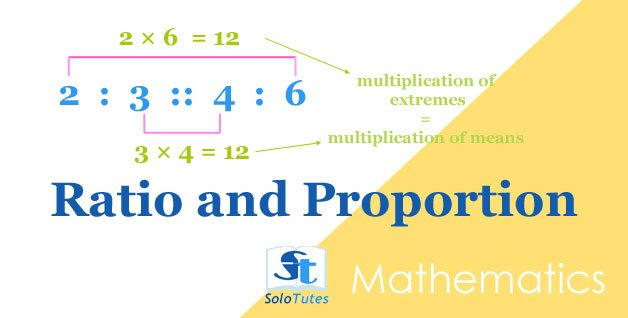SoloTutes

4 Posts

### All## Ratio and Proportion, Basic Tricks and Practice Problems## Practice Test #2 |  Pharmaceutics-II | MCQs for Pharmacy Exams## Practice Test #2 | Pharmaceutical Chemistry-II (Organic)## Practice Problems on Average | Math Quiz

1 of 3 〉 The average weight of A, B and C is  45 kg, that of A and B is 40 kg and B and C is 43 kg. What is the weight of B?
• 17 kg
• 26 kg
• 20 kg
• 31 kg
2 of 3 〉 The average of following 5 numbers is 8, find the missing number in 7, 5, 3,12,?.
• 14
• 13
• 8
• 12

1 more 👇

### Quiz / Practice Tests## Practice Test #2 |  Pharmaceutics-II | MCQs for Pharmacy Exams## Practice Test #2 | Pharmaceutical Chemistry-II (Organic)## Practice Problems on Average | Math Quiz

1 of 3 〉 The average weight of A, B and C is  45 kg, that of A and B is 40 kg and B and C is 43 kg. What is the weight of B?
• 17 kg
• 26 kg
• 20 kg
• 31 kg
2 of 3 〉 The average of following 5 numbers is 8, find the missing number in 7, 5, 3,12,?.
• 14
• 13
• 8
• 12

1 more 👇